RD Sharma Solutions - Ex-16.5, Congruence, Class 7, Math

# RD Sharma Solutions - Ex-16.5, Congruence, Class 7, Math - Notes | Study RD Sharma Solutions for Class 7 Mathematics - Class 7

 1 Crore+ students have signed up on EduRev. Have you?

#### Question 1:

In each of the following pairs of right triangles, the measures of some parts are indicated along side. State by the application of RHS congruence condition which are congruent. State each result in symbolic form. (Fig. 46)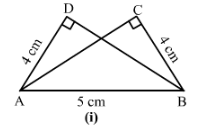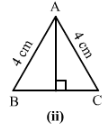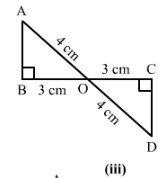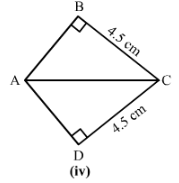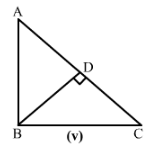i)
and hyp AB = hyp AB
.

ii)
hyp AC  = hyp AB (Given)

iii)
hyp AO = hyp DO
BO = CO
∠B =∠C =90°
Therefore, by RHS, △AOB≅△DOC

iv)
Hyp AC = Hyp CA
BC= DC

v)
BD = DB
Hyp AB =Hyp BC, as per the given figure.
∠BDA +∠BDC = 180°
∠BDA +90° = 180°
∠BDA= 180°−90°= 90°
∠BDA =∠BDC = 90°
Therefore, by RHS, △ABD≅△CBD

#### Question 2:

ABC is isosceles with AB = AC. AD is the altitude from A on BC.
(i) Is ∆ ABDACD?
(ii) State the pairs of matching parts you have used to answer (i).
(ii) Is it true to say that BD = DC?

(i)Yes, ABDACD△ABD≅△ACD by RHS congruence condition.
(ii) We have used Hyp AB = Hyp AC
(iii)Yes, it is true to say that BD = DC (c.p.c.t) since we have already proved that the two triangles are congruent.

#### Question 3:

ABC is isoseles with AB = AC. Also, ADBC meeting BC in D. Are the two triangles ABD and ACD congruent? State in symbolic form. Which congruence condtion do you use? Which side of ∆ ADC equls BD? Which angle of ∆ ADC equals ∠B?

We have AB = AC  ......(1)

Therefore from 1, 2 and 3, by RHS congruence condition,
△ABD△ACD

Now, the triangles are congruent .
Therefore, BD= CD.
And ∠ABD=∠ACD (c.p.c.t).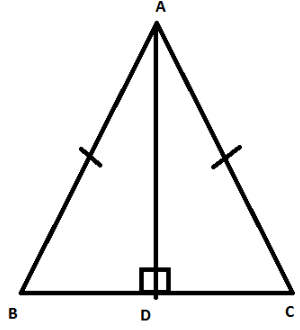#### Question 4:

Draw a right triangle ABC. Use RHS condition to construct another triangle congruent to it.

Consider
△ABC with ∠B as right angle.
We now construct another right triangle on base BC, such that    ∠C is a right angle and     AB = DC
Also, BC = CB
Therefore, by RHS, △ABC△DCB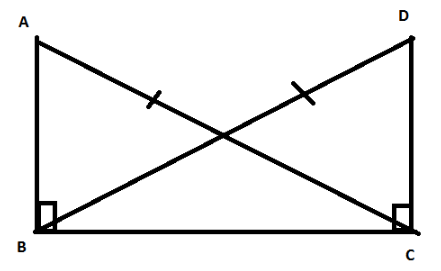#### Question 5:

In Fig. 47, BD and CE are altitudes of ABC and BD = CE.
(i) Is ∆ BCD ≅ ∆ CBE?
(ii) State the three pairs of matching parts you have used to answer (i).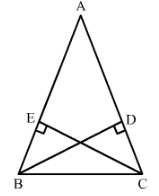(i) Yes, BCDCBE by RHS congruence condition.
(ii) We have used hyp BC = hyp CB
BD = CE (given in question)
and BDC = CEB =90°

The document RD Sharma Solutions - Ex-16.5, Congruence, Class 7, Math - Notes | Study RD Sharma Solutions for Class 7 Mathematics - Class 7 is a part of the Class 7 Course RD Sharma Solutions for Class 7 Mathematics.
All you need of Class 7 at this link: Class 7

## RD Sharma Solutions for Class 7 Mathematics

97 docs
 Use Code STAYHOME200 and get INR 200 additional OFF

## RD Sharma Solutions for Class 7 Mathematics

97 docs

Track your progress, build streaks, highlight & save important lessons and more!

,

,

,

,

,

,

,

,

,

,

,

,

,

,

,

,

,

,

,

,

,

,

,

,

,

,

,

,

,

,

;